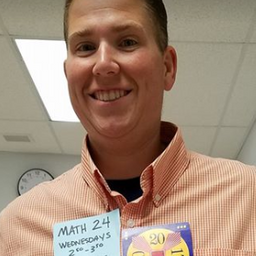# Prealgebra

## EducatorsSR
JT
+ 7 more educators

### Problem 1

In the following exercises, write as the ratio of two integers.
$$(a)5$$
$$(b)3.19$$

Check back soon!

### Problem 2

In the following exercises, write as the ratio of two integers.
$$(a)8$$
$$(b)-1.61$$Joshua F.

### Problem 3

In the following exercises, write as the ratio of two integers.
$$(a)-12$$
$$(b)9.279$$Stephen R.

### Problem 4

In the following exercises, write as the ratio of two integers.
$$(a)-16$$
$$(b)4.399$$Pratul K.

### Problem 5

In the following exercises, determine which of the given numbers are rational and which are irrational.

$$0.75,0.22 \overline{3}, 1.39174 \ldots$$

SR
Sophia R.

### Problem 6

In the following exercises, determine which of the given numbers are rational and which are irrational.

$$0.36,0.94729 \ldots, 2.528$$

Check back soon!

### Problem 7

In the following exercises, determine which of the given numbers are rational and which are irrational.

$$0 . \overline{45}, 1.919293 \ldots, 3.59$$

Check back soon!

### Problem 8

In the following exercises, determine which of the given numbers are rational and which are irrational.

$$0.1 \overline{3}, 0.42982 \ldots, 1.875$$

SR
Sophia R.

### Problem 9

In the following exerdises, identify whether each number is rational or irrational.
$$(a)\sqrt{25}$$
$$(b)\sqrt{30}$$

JT
John T.

### Problem 10

In the following exerdises, identify whether each number is rational or irrational.
$$(a)\sqrt{44}$$
$$(b)\sqrt{49}$$

Check back soon!

### Problem 11

In the following exerdises, identify whether each number is rational or irrational.
$$(a)\sqrt{164}$$
$$(b)\sqrt{169}$$

Check back soon!

### Problem 12

In the following exerdises, identify whether each number is rational or irrational.
$$(a)\sqrt{225}$$
$$(b)\sqrt{216}$$De'Sean K.

### Problem 13

In the following exercises, determine wheth number is whole, integer, rational, irrational, and real.
$$-8,0,1.95286 \ldots, \frac{12}{5}, \sqrt{36}, 9$$

Check back soon!

### Problem 14

In the following exercises, determine wheth number is whole, integer, rational, irrational, and real.
$$-9,-3 \frac{4}{9},-\sqrt{9}, 0.4 \overline{09} \cdot \frac{11}{6}, 7$$

Check back soon!

### Problem 15

In the following exercises, determine wheth number is whole, integer, rational, irrational, and real.
$$-\sqrt{100},-7,-\frac{8}{3},-1,0.77,3 \frac{1}{4}$$

Check back soon!

### Problem 16

Field trip All the 5 th graders at Lincoln Elementary School will go on a field trip to the science museum.
Counting all the children, teachers, and chaperones, there will be 147 people. Each bus holds 44 people.
(a)How many buses will be needed?
(b) Why must the answer be a whole number?
(c) Why shouldn't you round the answer the usual way?

HQ
Heba Q.

### Problem 17

Child care Serena wants to open a licensed child care center. Her state requires that there be no more
than 12 children for each teacher. She would like her child care center to serve 40 children.
(a) How many teachers will be needed?
(b) Why must the answer be a whole number?
(c) Why shouldn't you round the answer the usual way?

RB
Rebecca B.

### Problem 18

In your own words, explain the difference between a rational number and an irrational number.Will C.